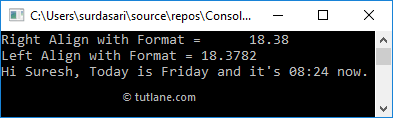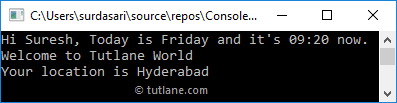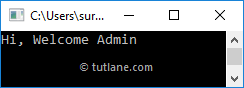# C# String Interpolation (\$)

In c#, String Interpolation is a feature that is used to embed any valid expression that returns a value into a string using `\$` operator. The string which is prepended with `\$` operator will call an interpolated string, and this feature is available from c# 6.0.

In c#, the String Interpolation is an extended version of the string.Format() feature to provide a more readable and convenient way to include formatted string expression results into a string literal.

For example, by using string.Format() method, we can insert the values in a string like {0}, {1}, etc. but by using String Interpolation, we can directly include any valid c# expression that returns a value in an interpolated string using braces like {name} or {expression}.

Following is the example of using both string.Format() and string interpolation in c# to include expression values inside of a string.

string name = "Suresh";
DateTime date = DateTime.Now;

// Normal formatting
string strFormat = string.Format("Hi {0}, Today is {1} and it's {2:HH:mm} now.", name, date.DayOfWeek, date);
Console.WriteLine(strFormat);
// Output: Hi Suresh, Today is Friday and it's 05:38 now.

// String interpolation
string strIntr = \$"Hi {name}, Today is {date.DayOfWeek} and it's {date:HH:mm} now.";
Console.WriteLine(strIntr);
// Output: Hi Suresh, Today is Friday and it's 05:38 now.

If you observe the above examples, we used a string.Format() method with {0}, {1}, {2} to embed values in string but with String Interpolation we directly included a valid c# expressions within the string.

## C# String Interpolation Structure

In c#, to identify a string literal as an interpolated string, we need to prepend it with `\$` symbol, and there should not have any whitespace between the `\$` symbol and the double quote `"` that starts a string literal.

Following is the structure of interpolated string with required expressions in c# programming language.

{<interpolatedExpression>[,<alignment>][:<formatString>]}

Here the elements in square brackets `[]` are optional. The alignment and formatString elements are useful to format and adjust the alignment of the interpolated string based on our requirements.

The following table lists the details about each element interpolated strings in c#.

ElementDescription
interpolatedExpression The expression that produces a result and it will be included in the interpolated string with braces {}
alignment It's a constant expression, and it is used to specify an alignment for expression result using comma(,). If the alignment value is positive, then the formatted expression result is right-aligned; if negative, it's left-aligned.
formatString A format string that is supported by the type of expression result. To format the given expression, we can define formatString with interpolated expression using the colon (":").

Following is the example of using optional formatting components in the interpolated string.

double x = 12.34562;
double y = 6.032592;
const int ealign = 10;
Console.WriteLine(\$"{x} + {y} = {x + y}");
Console.WriteLine(\$"Sum with Format = {x+y, ealign:F2}");

// Output:
// 12.34562 + 6.032592 = 18.378212
// Sum with Format = 18.38

If you observe the above example, we used optional formatting components to format the expression. Using the alignment element (ealign), we made the expression result is right-aligned, and by using the format string (F2) we limited the expression result to 2 decimal points.

## C# String Interpolation Example

Following is the example of creating string interpolation strings in c#.

using System;

namespace TutlaneExamples
{
class Program
{
static void Main(string[] args)
{
string name = "Suresh";
DateTime date = DateTime.Now;
double x = 12.34562;
double y = 6.032592;
const int ealign = 10;
Console.WriteLine(\$"Sum of {x} + {y} = {x + y}");
Console.WriteLine(\$"Right Align with Format = {x + y,ealign:F2}");
Console.WriteLine(\$"Left Align with Format = {x + y,-10:F4}");
string strIntr = \$"Hi {name}, Today is {date.DayOfWeek} and it's {date:HH:mm} now.";
Console.WriteLine(strIntr);
}
}
}

If you observe the above example, we defined the interpolated strings using dollar (`\$`) character, specified alignment, and format based on our requirements.

When we execute the above c# program, we will get the result as shown below.If you observe the above result, the interpolated expression results are aligned right or left based on the alignment value, and decimal points are also limited based on the defined format.

## C# Multiline String Interpolation

In c#, the String Interpolation will support multiline or verbatim interpolated string, which starts with ‘`@`’ character. To implement the verbatim interpolated string, the string must start with `\$` character followed by `@` character.

Following is the example of implementing a multiline or verbatim interpolated string in c#.

using System;

namespace TutlaneExamples
{
class Program
{
static void Main(string[] args)
{
string name = "Suresh";
DateTime date = DateTime.Now;
string msg = \$@"Hi {name}, Today is {date.DayOfWeek} and it's {date:HH:mm} now.
Welcome to Tutlane World
Console.WriteLine(msg);
}
}
}

If you observe the above example, we implemented a multiline interpolated string using `\$` character and it is followed by `@` character.

When you execute the above c# program, you will get the result as shown below.This is how we can implement a multiline interpolated string in the c# programming language based on our requirements.

## C# String Interpolation with Ternary Operator (?:)

In c#, we can use conditional ternary (?:) operator in interpolated expressions by enclosing it in parenthesis `{}` because in string interpolation, the character colon  (“`:`”) is used to define the format for interpolation expression.

Following is the example of using conditional ternary (?:) operator in c# string interpolated expression.

using System;

namespace TutlaneExamples
{
class Program
{
static void Main(string[] args)
{
string name = "Suresh";
string msg = \$@"Hi, Welcome {(name == "Suresh" ? "Admin" : "Guest")}";
Console.WriteLine(msg);
}
}
}

If you observe the above example, we defined a conditional ternary (?:) operator in interpolated expression with parenthesis `{}`.

When we execute the above c# program, we will get the result as shown below.This is how we can use conditional ternary (?:) operator in string interpolation based on our requirements.

## C# String Interpolation Performance

In c#, the string interpolation performance is almost same as String.Format() method. There is a tiny bit of overhead for the string interpolation in c# due to expressions embedding in the string, but that is very, very small.

From a performance point of view, both string interpolation and string.Format() are not suggestible. Instead, you can use StringBuilder. This is how we can use string interpolation in c# to include valid expressions within the string based on our requirements.